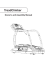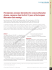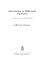# THE SHADOW-CURVES OF THE ORBIT DIAGRAM PERMEATE

## Transcription

THE SHADOW-CURVES OF THE ORBIT DIAGRAM PERMEATE
```October 12, 2009
13:45
02462
International Journal of Bifurcation and Chaos, Vol. 19, No. 9 (2009) 3017–3031
c World Scientiﬁc Publishing Company
THE SHADOW-CURVES OF THE ORBIT DIAGRAM
PERMEATE THE BIFURCATION DIAGRAM, TOO
CHIP ROSS
Department of Mathematics,
Bates College, Lewiston, ME 04240, USA
MEREDITH ODELL
12 Meter Charters,
49 Bowen’s Wharf, Newport, RI 02840, USA
SARAH CREMER
Research Assistant, WestEd,
400 Seaport Court, Suite 222,
Redwood City, CA 94063, USA
Received April 2, 2007; Revised February 27, 2009
The “Q-curves” Q1 (c) = c, Q2 (c) = c2 + c, . . . , Qn (c) = (Qn−1 (c))2 + c = fcn (0) have long been
observed and studied as the shadowy curves which appear illusively — not explicitly drawn —
in the familiar orbit diagram of Myrberg’s map fc (x) = x2 + c. We illustrate that Q-curves also
appear implicitly, for a diﬀerent reason, in a computer-drawn bifurcation diagram of x2 + c as
well — by “bifurcation diagram” we mean the collection of all periodic points of fc (attracting,
indiﬀerent and repelling) — these collections form what we call “P -curves”. We show Q-curves
and P -curves intersect in one of two ways: At a superattracting periodic point on a P -curve, the
inﬁnite family of Q-curves which intersect there are all tangent to the P -curve. At a Misiurewicz
point, no tangencies occur at these intersections; the slope of the P -curve is the ﬁxed point of
a linear system whose iterates give the slopes of the Q-curves.
We also introduce some new phenomena associated with c sin x illustrating brieﬂy how its
two diﬀerent families of Q-curves interact with P -curves.
Our algorithm for ﬁnding and plotting all periodic points (up to any reasonable period) in
the bifurcation diagram is reviewed in an Appendix.
Keywords: Myrberg map; orbit diagram; bifurcation diagram; attracting, repelling, and indifferent periodic point; preperiodic point; Q-curve; P -curve; Misiurewicz point.
1. Introduction
across each of them. We call these Q-curves, and
although they can be explicitly plotted (see Fig. 3)
using Myrberg’s recurrence formulas
1.1. Q-curves and P -curves
Like the well-known orbit diagram of Fig. 1, a
computer-generated bifurcation diagram (Fig. 2) for
Myrberg’s map fc (x) = x2 + c has fascinating features worthy of study. One striking feature shared
by these two self-similar diagrams is the illusion of
shadowy polynomial curves sweeping up and down
Q1 (c) = c,
Q2 (c) = c2 + c, . . . , Qn (c)
= (Qn−1 (c))2 + c = fcn (0), (1)
they are not explicitly drawn in either the orbit
or the bifurcation diagram, and in fact appear
implicitly in both for very diﬀerent reasons.
3017
October 12, 2009
3018
13:45
02462
C. Ross et al.
Fig. 1.
Familiar Orbit diagram.
Myrberg  was the ﬁrst to study properties
of Q-curves, and in particular, was interested in
ﬁnding relationships among their roots and accumulations points of these roots. Using Myrberg’s
results, Mira  further studied these accumulation points and their relationships with intersecting
families of Q-curves. The details of why Q-curves
can be seen in the orbit diagram, and the slopes of
Q-curves at points where they intersect, are given in
[Neidinger & Annen, 1996]; brieﬂy, we see Q-curves
in Fig. 1 because chaotic orbits of 0 “pile up” more
above than below Q-curves (or vice versa) and the
abrupt diﬀerence in densities of points above versus
below makes the Q-curves visible.
Our contribution to this topic begins with
the observation that Q-curves also appear implicitly in a true bifurcation diagram such as in
Fig. 2. This picture, drawn using our algorithm1 in
1
Fig. 2.
Detailed Bifurcation diagram.
[Ross & Sorensen, 2000] shows all periodic points
(up through period 12 here), not just the attracting ones seen in the orbit diagram. The periodic
points themselves form curves having very elongated “⊃”-shapes which we shall call “P -curves”.
These P -curves all reach in from the left side of the
ﬁgure, turn around (at all the various c’s for which a
and exit left again.
Figure 3 shows several Q-curves, explicitly
drawn using the formulas in (1). All three Figs. 1–3
illustrate that Q-curves intersect one another in
one of two distinctly diﬀerent ways: At any such
intersection, either all Q-curves are tangent to one
another (for example, at the point T in Fig. 3), or
else no two are tangent (as at M ). Several of each of
the intersections can be seen in Fig. 3. Proving this
dichotomy is a main topic in [Neidinger & Annen,
Brieﬂy, the algorithm seeks crossings of iterates of f and the line y = x. It does not use “backwards iteration”, nor does it
attempt solving f n
c (x) = x for x in terms of c. A brief description of the algorithm is provided in the Appendix.
October 12, 2009
13:45
02462
Shadow-Curves Permeate the Bifurcation Diagram, Too
3019
Fig. 3. The ﬁrst six Q-curves, colored to make them easier
to distinguish. We will study Q- and P -curve interactions at
c = γ. (The choice of colors is independent of the next ﬁgure.)
Fig. 4. P - and Q-curve interactions. Points on P -curves are
colored according to their prime period; Q-curves are black.
The asterisk marks the speciﬁc P - and Q-curve intersections
studied in detail in Fig. 8.
1996]. Our purpose is to show how this dichotomy
extends to the ways the Q-curves interact with P curves; we illustrate this in Fig. 4 as follows.
Figure 4 shows P -curves through prime
period 6 in color and Q1 through Q8 in black. Now,
we expect to see that a P -curve passes through any
intersection of Q-curves (when such an intersection
occurs at some c, the critical point 0 itself is either
a periodic or eventually periodic point for fc ; we
review this below). But we observe that at any such
point where Q-curves are all tangent, the P -curve
looks tangent to all the Q-curves. Several of these
mutually tangent intersections of P - and Q-curves
can be seen (a few are marked “t” and there are ﬁve
along the dotted line; a close-up of the one at ∗ is
oﬀered in Fig. 8). Note such intersections are near
but not at the tip of the involved P -curve (since
for c at the tip the slope of f nc is 1 as the bifurcation occurs; after a small decrease in c this slope
becomes 0 and gives the Q-curve intersection). The
Q-curves are lined with such intersections as the
bifurcations develop from c = 0.25 down to c = −2
and so become visible in Fig. 2 because of the “density diﬀerence” as there are more P -curves on the
left than on the right of the Q-curves.2
A mutual tangency is one of the two distinct ways the bifurcation diagram’s explicitlydrawn P -curves interact with Q-curves. The other
interaction is not so obvious, but is dramatic: Wherever Q-curves cross and none are tangent, the corresponding P -curve is not tangent to any Q-curve
there (two such crossings are labeled “s”). In fact,
2
The development of these bifurcations follows a speciﬁc pattern, as studied for example by Mira  using his “box-withina-box” paradigm.
October 12, 2009
3020
13:45
02462
C. Ross et al.
the slope of the P -curve is the ﬁxed point value of
a simple linear system which when iterated starting
with the slope of the lowest-numbered Q-curve in
the intersection, produces the slopes of all remaining Q-curves at the intersection. (This is really
what also happens in the ﬁrst kind of intersection,
but that simple system reduces to an even simpler
constant system.) Developing the formula for these
Remark. In Fig. 4 there are intersections visible
where a P -curve and a single Q-curve meet. We
can always see that more Q-curves meet at such a
point by just plotting enough of them as there are
inﬁnitely many Q-curves at any such intersection.
However, for each additional Q-curve (or higherorder P -curve) we plot, the picture gains phenomenally more points where a P -curve seems to meet
but one Q-curve.
1.2. Orbit diagrams versus
bifurcation diagrams
The orbit diagram of Fig. 1 will be familiar to all
readers; indeed it “has become the most important icon of chaos theory” [Peitgen et al., 1992].
For each c in a range of c-values on the horizontal axis, it plots on the vertical axis the longterm behavior of the orbit of 0 for the function
fc (x) = x2 + c. Such long-term behavior reveals
intervals of c values in which fc has an attracting cycle and suggests chaotic behavior at other c
values. However, the orbit diagram is often called
the “bifurcation diagram”.3 But we shall follow the
notation of Peitgen et al. , Devaney ,
and Strogatz , and reserve that name for the
diagram in Fig. 2. This genuine bifurcation diagram
plots all the periodic points (up to period 12 in
this particular ﬁgure) of fc — not just those on
attracting cycles, but also those on repelling cycles;
repelling cycles do not appear in the familiar orbit
diagram. Note that both diagrams show perioddoubling bifurcations, as attracting cycles “split”
at certain c-values, but the bifurcation diagram
also shows how the repelling cycles persist even
as c decreases through such bifurcations — compare both ﬁgures in the region c > −1.401, for
example, where fc undergoes the familar 1–2–4–8–
· · · period-doubling scheme. Additionally, because
it shows both attracting and repelling points, the
3
bifurcation diagram also gives a more complete picture of what happens at saddle-node bifurcations
(look ahead to Fig. 7, for example). Indiﬀerent periodic points appear in both diagrams implicitly, as
they are the periodic points found right at either
kind of bifurcation.
1.3. Notation and basic definitions
Let f be one of the members of the family fc (x) =
x2 + c obtained by ﬁxing a speciﬁc value of c. The
orbit of a point x under f is the sequence x, f (x),
f 2 (x), f 3 (x), . . . , of iterates of x; here f n (x) means
the composition of f with itself n-times. A real number p is a point of period n for f if f n (p) = p. If n is
the smallest positive integer for which this equality
holds, we say p has prime period n, and if n = 1 we
call p a ﬁxed point. If p is a point of prime period
n for f , then the n iterates p, f (p), . . . , f n−1 (p) are
called the n-cycle to which p belongs. If |(f n ) (p)| <
1, the cycle is called an attracting (or stable) cycle
(and super attracting if (f n ) (p) = 0); this is
because one can prove the orbits of all points close
to p approach the orbit of p. The orbit diagram
shows attracting cycles for fc (x) = x2 + c because
if there is such a cycle for a given c, this cycle must
attract the orbit of the critical point 0. However the
orbit diagram shows many c-values for which the
orbit of 0 appears as a sequence of scattered points,
suggesting no attracting cycle exists and instead, fc
exhibits chaotic behavior.
Again let p have prime period n. Now if
|(f n ) (p)| > 1, the n-cycle is called repelling (or
unstable); in this case, there is some interval (a, b)
containing p such that for any x ∈ (a, b) with x = p,
the orbit of x moves away from the orbit of p.
Finally, if |(f n ) (p)| = 1, we will simply say the cycle
is indiﬀerent. In any case — no matter the value of
|(f n ) (p)| — the bifurcation diagram contains the
point (c, p).
Finally, in Fig. 4, we see two ways that Q-curves
intersect: At any such intersection, either all Qcurves are tangent, or else not even a pair of them
are tangent. We refer to these as intersections of the
ﬁrst and second kinds, respectively.
2. Primitive Zeros and Intersections
of the First Kind
Following Myrberg, we say r is a primitive zero of
Qn if Qn (r) = 0 and Qj (r) = 0 for 0 < j < n. Since
In particular, in [Neidinger & Annen, 1996], the orbit diagram is called the bifurcation diagram.
October 12, 2009
13:45
02462
Shadow-Curves Permeate the Bifurcation Diagram, Too
his work may be somewhat unsung, we quickly mention some pertinent results from Myrberg .
Noting that if r is a speciﬁc primitive zero of
Qn , Myrberg showed that r satisﬁes a “radical
equation”
√ ···
−r
(2)
r = −−r ± −r ± · · · ±
(1)
(2)
(n−2)
consisting of n − 1 radical signs and a speciﬁc
“rotation sequence” of “+” and “−” signs in place
of the n − 2 “±” signs in (2); Myrberg’s ordering law shows how the relative positions of primitive zeros can be ascertained from their respective
rotation sequences. Furthermore, for those rotation sequences for which (2) admits a real solution r, Myrberg produced a table of all primitive
zeros for Qn , 3 ≤ n ≤ 10; the table has some 120
entries all calculated to 10 decimal places. (Our
illustration in Sec. 2.3 considers the primitive zero
γ ≈ −1.6254137251 of Q5 ; the rotation sequence
for γ is (+ − −).) Myrberg developed a formula
for the number of primitive zeros of Qn , which is
thus a count of the total number of saddle node
and period-doubling bifurcations of period n which
occur in [−2, 0.25]. Indeed, through his study of
primitive zeros, Myrberg discovered the “perioddoubling” phenomena and was the ﬁrst to identify Myrberg–Feigenbaum points, which are the
limit points of such sequences. For example, by
computing numerical values of the rightmost primitive zeros of Q2k for 1 < k ≤ 9, he determined they converged to a value between −1.40117
and −1.40115; today −1.401155189 is often called
“the” (Myrberg) Feigenbaum point. Myrberg was
aware that there are inﬁnitely many such points in
[−2, 0.25] for the family x2 + c. For a complete discussion of Myrberg’s results and their consequences,
see [Mira, 1987].
Myrberg noted that primitive zeros of Qn correspond to superattracting periodic points of f , and
Neidinger and Annen  discussed the slopes
of Q-curves as they intersect at primitive zeros.
Explicitly, we have:
2.1. Intersection dichotomy part 1
If r is a primitive zero of Qn , then 0 is on a superattracting cycle of prime period n for fr (x) = x2 + r.
All Q-curves of the form Qj+sn meet and are tangent at the point (r, f j (0)), where 0 < j ≤ n, for
s = 0, 1, 2, . . . .
3021
That is, let pj = f jr (0) be the jth point in
the periodic cycle f r (0), fr2 (0), . . . , f nr (0) = 0 =
Qn (r). The curve Qj and every nth Q-curve thereafter meet and are tangent at (r, pj ). In particular,
Q1 , Q1+n , Q1+2n , . . . , all meet and are tangent at
(r, r), while Qn , Qn+n , Qn+2n , . . . , all meet and are
tangent at (r, 0).
2.2. Our extension of the
intersection dichotomy part 1
to the slopes of P -curves
At r these n families of Q-curves are also tangent to
the corresponding P -curves of the bifurcation diagram which pass through those n common intersections. Speciﬁcally, Pn,j is tangent to all the Q-curves
Qj+sn at r, where for 0 < j ≤ n, Pn,j is the top or
bottom “half” of the P -curve containing the point
(r, pj ).
We remark that for any n > 3 there are at least
two separate families of P -curves of points of prime
period n. Indeed Myrberg  and Mira 
give formulas which count the number of families of
P -curves of a given prime-period, and give laws for
the order in which they appear from left to right.
Fortunately, the ordering does not enter into the
slope calculation. For our purposes, of the many
curves which could be named Pn,j , Pn,j refers to
a unique curve once the speciﬁc P -curve is identiﬁed as the one to which the prime period n point
pj = f jr (0) belongs. On the other hand, for each n,
Qn is a unique curve.
2.3. Illustration
We will prove the extension later (Sec. 3.3). Let c =
γ ≈ −1.6254. Figure 3 shows that Q1 (γ), . . . , Q5 (γ)
are all distinct and Q5 has a root at γ, so fγ (0),
f 2γ (0), f 3γ (0), f 4γ (0), and f 5γ (0) = 0 form a prime
period 5-cycle for fγ . In Fig. 5, the graph of f 5γ
intersects id (the line y = x) 12 times. Now, in the
boxes are two “steep” crossings representing the two
ﬁxed points for fγ as the parabola fγ also crosses
id at those two points. But our concern is the ten
intersections near the local max/mins; these occur
in very close pairs, one of which is circled. Each pair
contains one point representing one of the members
of the above 5-cycle; the other ﬁve points in the
pairs belong to another 5-cycle. We “zoom in” to
the circled pair: In Fig. 6, G ≈ (−0.6085, −0.6085)
and Γ ≈ (−0.5920, −0.5920) are on f 5γ ; −0.5920 is
f 3γ (0) and −0.6085 is on the other 5-cycle. Corresponding to these two points of prime period 5, the
October 12, 2009
3022
13:45
02462
C. Ross et al.
Fig. 5. There are 12 intersections of f 5γ with id, the line
y = x. The circled region, which contains two of the 12, is
enlarged in Fig. 6.
Fig. 7. Part of the bifurcation diagram, corresponding to
Fig. 6, showing points of prime periods 5, 10 and 20. Points
on attracting cycles are orange, repelling periodic points are
in blue and indiﬀerent periodic points are found where the
two colors meet.
Fig. 6. Four similar curves yielding diﬀerent dynamics: they
show fα has no points of prime period 5, fβ has a single, indifferent prime period 5-cycle, fγ has two prime period 5-cycles,
one repelling, the other superattracting, and fδ also has two,
one repelling and one indiﬀerent. At this scale, Fig. 5 would
be over 17 feet wide.
portion of the bifurcation diagram shown in Fig. 7
contains Ĝ = (γ, −0.6085) and Γ̂ = (γ, −0.5920).
Part 1 of the intersection dichotomy and its extension say that starting with Q3 , every ﬁfth Q-curve is
tangent to the P -curve P5,3 at Γ̂, and this is illustrated in Fig. 8 at ∗. We note in passing that Γ̂
would appear on the orbit diagram, but Ĝ would
not; the slope of f 5γ at G in Fig. 6 is clearly greater
than one so −0.6085 is on a repelling 5-cycle. Also,
we have focused on just one member f 3γ (0) of the
5-cycle; four other pictures similar to Fig. 8 can be
drawn for each of the other members of the cycle at
their respective places on the bifurcation diagram.
Finally, note there are some unlabeled repelling
periodic points in the upper right corners of Figs. 7
and 8; from the top these are points of prime periods 18, 20, 19 and 17. Tiny portions of the bottom
halves of three of these P -curves can be seen in the
lower right corners of these ﬁgures.
In Fig. 7, note that Γ̂ is near, but not at, the
tip of P5,3 . The tip itself is at Ŝ ≈ (β, −0.5998),
October 12, 2009
13:45
02462
Shadow-Curves Permeate the Bifurcation Diagram, Too
Fig. 8. Q-curves and the bifurcation diagram. The asterisk
at Γ̂ marks the same point in both Figs. 4 and 8. At this
point, Q3 , Q8 , Q13 , . . . , are all tangent to P5,3 .
where c = β ≈ −1.6244 is the value at which f 5c
undergoes a saddle-node bifurcation: For c > β, fc
has no points of prime period 5. Such is the case
with fα (α ≈ −1.6220): in Fig. 6, f 5α has those ﬁve
similar max/mins, but they do not cross id. When c
decreases to β, f 5β becomes tangent to id right near
each of the ﬁve local max/mins simultaneously —
one of these points is S ≈ (−0.5998, −0.5998) in
Fig. 6. Thus fβ has a single prime period 5-cycle.
As c decreases further, f 5c crosses id twice near each
of the max/mins, yielding two sets of prime period
5-cycles of fc , one repelling and the other attracting.
Importantly, only a small decrease in c, from β
to γ, puts one intersection in Fig. 6 of f 5c and id right
at the max/min itself. The corresponding 5-cycle is
4
3023
superattracting — the derivative of f 5c at this intersection is 0, and so by the chain rule, 0 itself is part
of the cycle; this aﬃrms that this max/min crossing occurs when c = γ, and illustrates why Q-curves
are tangent to P -curves just to the left of the tips of
saddle-node bifurcations, where (after “only a small
decrease in c”) the superattracting periodic points
are found. Figures 2 and 4 are loaded with such
saddle-node bifurcations, and a family of Q-curves
is tangent to a point near the tip of every one of
them.
P -curves resulting from period-doubling bifurcations also abound in Fig. 2, but most are in
places where the diagram is quite crowded.4 Yet
two families of Q-curves are tangent to two points
on superattracting cycles near the tip of every such
P -curve. We will illustrate this by developing our
example further. Points of prime period 5 are points
of period 10. As c drops below δ ≈ −1.6284,
f 10
c begins to twist around enough to cross id in
two new places on each side of each point on the
attracting 5-cycle. A typical period-doubling bifurcation, these ten new crossings give fc a single, new
attracting prime period 10-cycle, while the 5-cycle
becomes repelling. The intersection of f 5δ and id at
D ≈ (−0.5853, −0.5853) in Fig. 6 shows fδ has a
5-cycle containing −0.5853. There the slope of f 5δ is
−1 and the changeover from attracting to repelling
occurs as c decreases through δ. Figure 9 shows a
zoom of f 10
δ also at D — the period-doubling is
imminent and is marked by D̂ ≈ (δ, −0.5853) in
Fig. 7. In Fig. 10 the zoom now shows f 10
c after
only a slight decrease in c to c = µ ≈ −1.6294.
Here the extrema are points of prime period 10
for fµ ; again by the chain rule, 0 itself is on this
Fig. 9. A period-doubling bifurcation is imminent when
c = δ ≈ −1.6284.
Because it shows only prime periods from 1 to 6, just two period doubling bifurcations occur in Fig. 4 — the prime period
2-to-4, and the 3-to-6. Adding more P -curves to see more period doubling bifurcations would crowd an already very dense
picture.
October 12, 2009
3024
13:45
02462
C. Ross et al.
P10,3 and P10,8 at M̂3 and M̂8 with the two families
Q3 , Q13 , Q23 , . . . and Q8 , Q18 , Q28 , . . . , respectively.
The Q-curves thus undergo a kind of bifurcation
of their own, as (from right to left) they split oﬀ
from Γ̂ alternately heading up or down to meet
at M̂3 or M̂8 . This “Q-curve bifurcation” happens
again at M̂8 , where Q18 moves up to P20,18 , while
Q8 and Q28 head down and are tangent again to
P20,8 at .
Fig. 10. Shortly after the bifurcation when c = µ ≈
−1.6294, the max/mins are on the cycle, yielding a superattracting prime period 10 cycle for fµ .
3. Intersections of the Second Kind
If Q-curves meet at some c = s which is not a root
of any Q-curve, it follows that 0 is not a periodic
point for fs , but its orbit does become periodic,
superattracting 10-cycle and Q10 has a root at
with prime period determined by the smallest diﬀerµ. Indeed, the third and eighth iterates f 3µ (0) ≈
ence between the subscripts of any pair of Q-curves
−0.5774 and f 8µ (0) ≈ −0.5941 appear in Fig. 10 at
which intersect at s. In this case, we say the orbit
of 0 is strictly preperiodic and refer to s as a
M3 and M8 . Correspondingly, Fig. 7 contains M̂3 ≈
Misiurewicz point.
(µ, −0.5774) and M̂8 ≈ (µ, −0.5941). (The repelling
Let k and n be the smallest positive integers for
period 5 point at R in Fig. 10 also appears at R̂ in
which
Qk and Qk+n intersect at s. The orbit of 0
Fig. 7.) Figure 8 shows the expected tangency of
has the form
k+n−1
k+n
k+2n
(0),
.
.
.
,
f
(0),
f
(0),
.
.
.
,
f
(0),
.
.
.
,
.
.
.
0, fs (0), . . . , f ks (0), f k+1
s
s
s s no repeats
prime period n-cycle
Note that Q-curves Q1 , Q2 , . . . , Qk−1 , can have
no intersections with any other Q-curves nor P curves at s. For 0 ≤ j < n − 1, let Pn,j be the top or
bottom “half” of the P -curve containing (s, f k+j
s (0)).
From [Neidinger & Annen, 1996] we have:
3.1. Intersection dichotomy part 2
Suppose that for c = s, 0 is strictly preperiodic,
with k and n as above. Let 0 ≤ j < n − 1. Although
for any a, b ≥ 0 the Q-curves Qk+j+an and Qk+j+bn
intersect at (s, fsk+j (0)), they are never tangent, i.e.
no two intersecting Q-curves can be tangent at such
a point s.
Figure 4 suggests that at a Misiurewicz point,
the slope of the P -curve is diﬀerent from the slopes
of the Q-curves meeting there. However, the slope
of that P -curve has a fundamental relationship to
the slopes of those Q-curves:
3.2. Our extension of the
intersection dichotomy part 2
to the slopes of P -curves
As it passes through the intersection of the family of
Q-curves Qk+j+an (a = 0, 1, 2, 3, . . .), Pn,j is tangent
n-cycle
n-cycle
to none of them. At c = s, the slopes Dk+j+an of the
Q-curves Qk+j+an , a = 0, 1, 2, . . . are the iterates of
Dk+j for a simple linear function y = mx + bj . The
slope of Pn,j is the ﬁxed point of this function.
3.3. Proof of the tangency claims
We prove both extensions simultaneously by analyzing the behaviors of two linear systems. Suppose Q-curves intersect at some c = c. Let k be
the smallest non-negative integer, and n the smallest positive integer for which f ck (0) = f ck+n(0). If
k = 0 then c is a root of Qn and we are considering Q-curve intersections of the ﬁrst kind. If
k > 0 then c is a Misiurewicz point and the intersections are of the second kind. In the arguments
which follow, if k = 0 we take 0 < j ≤ n (so
that Qk+j cannot mean Q0 ), whereas if k > 0 we
take 0 ≤ j < n (so that Qk+j can represent Qk by
taking j = 0).
Let us write Dh for Qh , the derivative of Qh
(c) to mean the slope of
with respect to c, and Pn,j
Pn,j at the point (c, Qk+j (c)) where Pn,j and Qk+j
meet.
October 12, 2009
13:45
02462
Shadow-Curves Permeate the Bifurcation Diagram, Too
Proposition 1. Let k, n and j be as above. For
3025
D0 and Q0 deﬁned as 0 for convenience, we have
Dh+1 = 2Qh Dh + 1 and in general (with empty
products equal to 1),
each j, the slope values Dk+j+an (c), a = 0, 1, 2, . . .
are the iterates of Dk+j (c) for the linear function
y = mx + bj where m is the product of 2n and
the n members of the prime period n-cycle, and bj
is a combination (given in Eq. (3)) of these cycle
members which depends on j. The ﬁxed point of
(c).
y = mx + bj is the value Pn,j
In particular, if k = 0 then Dk+j+an (c) =
(c) = b for a = 0, 1, 2, . . .. If k > 0 the valPn,j
j
ues Dk+j+an (c) are pairwise unequal, and diﬀerent
(c).
from Pn,j
Dh+t = 2t Qh Qh+1 · · · Qh+t−1 Dh
+ 2t−1 Qh+1 · · · Qh+t−1 + · · ·
(3)
+ 4Qh+t−2 Qh+t−1 + 2Qh+t−1 + 1


t−1
u
t−1
Qh+p Dh +
2u
Qh+t−p . (4)
= 2t
To prove the proposition, we ﬁrst ﬁnd a recursive formula for the slopes of the Q-curves. With
By Eq. (4) the slope of Qk+j+an can be used to
ﬁnd the slope of the next higher numbered Q-curve
Q(k+j+an)+n which meets at (c, Qk+j (c)):

D(k+j+an)+n
= 2n
n−1
p=0

Q(k+j+an)+p  Dk+j+an +
p=0
n−1
u=0
u
2
u=0
u
p=1
Q(k+j+an)+n−p .
p=1
By periodicity, Qh (c) = Qh+an (c) for h ≥ k + j, so when the above expression is evaluated at c we obtain


n−1
u
n−1
n
u


Q(k+j)+p (c) · Dk+j+an (c) +
2
Q(k+j)+n−p (c) .
(5)
D(k+j+an)+n (c) = 2
p=0
u=0
p=1
“bj ”
“m”
For any j, the product in front of Dk+j+an (c) is 2n times (in some order) all n iterates of the cycle, and
we will call this expression m. The summation which follows is not independent of j and we will call it bj .
We have
D(k+j+an)+n (c) = mDk+j+an (c) + bj
for a = 0, 1, 2, . . . ,
(6)
that is, the slopes of the jth family of Q-curves intersecting at c are produced by iterating the function
y = mx + bj , starting at x = Dk+j (c).
for this derivative. In general,
A formula for dx/dc at (c, Qk+j (c)) on Pn,j is required. We write Pn,j
n
if the point (c, x) is on Pn,j then fc (x) = x. Implicit diﬀerentiation of this equation with respect to c and
solving for dx/dc yields

 

u
n−1
n−1
(c, x) = 
2u
fcn−p (x) ÷ 1 − 2n
fcp (x).
Pn,j
u=0
p=1
p=0
(c), is
In particular, the slope of Pn,j at (c, Qk+j (c)), that is, Pn,j
n−1
u
2
u=0
1−
u
n−1
fcn−p (fck+j (0))
p=1
n−1
p k+j
2n
fc (fc (0))
p=0
=
u=0
u
u
2
Q(k+j)+n−p (c)
p=1
n−1
n
1−2
If k = 0 (the ﬁrst kind of intersection) then
Qn (c) = 0 is one of the factors used to deﬁne m,
so m = 0. Equation (6) becomes Dj+an (c) = bj
for a ≥ 1, independently of the “ﬁrst” value Dj (c).
However, the upper limit n−1 of the summation for
=
Q(k+j)+p (c)
bj
.
1−m
(7)
p=0
bj in Eq. (5) can be replaced by j − 1, because for
u ≥ j, the factor p = j in the corresponding product
is Qn (c) = 0; also in Eq. (5), Qj+n−p(c) = Qj−p (c)
because of periodicity n. This and Eq. (4) with
October 12, 2009
3026
13:45
02462
C. Ross et al.
h = 0 and t = j show directly that Dj (c) = bj .
(c) = b also.
Finally m = 0 in Eq. (7) gives Pn,j
j
Thus for each j, 0 < j ≤ n, the Q-curves Qj+an ,
a = 0, 1, 2, . . . , are all tangent to the P -curve Pn,j
at c and their common slope is bj .
If k > 0 then because c is a Misiurewicz point,
the periodic cycle 0 eventually joins is repelling
[Carleson & Gamelin, 1993]. The chain rule shows
that if p is any member of that cycle, then m =
f cn (p); because the cycle is repelling, |m| > 1. Thus
the linear system y = mx + bj has but one peri (c).
odic point, the ﬁxed point bj /(1 − m) = Pn,j
Neidinger and Annen  show that Dk+j (c) =
Dk+j+n (c), so Dk+j (c) is not the ﬁxed point of
y = mx + bj and hence its orbit is made up of
distinct values, giving a concrete argument that no
two Q-curves are tangent at c, and none of them
have the same slope bj /(1 − m) as the P -curve
through (c, Qk+j (c)). In summary, the slope of Pn,j
at (c, Qk+j (c)) is the ﬁxed point of the linear system which produces the all-diﬀerent slopes of the
Q-curves through that point. The slope of Pn,j is
not equal to that of any of the Q-curves — in some
sense Pn,j “sits in the middle” while the Q-curves
get steeper and steeper around it.
at f 3c (0); in the notation above we have n = 2 and
k = 3. Therefore, Q-curves Q3 , Q5 , Q7 , . . . , all pass
through (c, 0.5437), while Q-curves Q4 , Q6 , Q8 , . . . ,
meet at (c, −1.5437); this second group of curves
is shown in Fig. 11. Using Dh+1 = 2Dh Qh + 1,
we calculated the slope of Q4 iteratively starting with Q 1 (c) = 1. The values m = −3.3571
and b1 = 2.0874 were found using Eq. (5). In
Fig. 11, the ratio of the vertical to the horizontal
scales is 100; the calculated values look reasonable,
and in fact, they are the same (to several decimal places) as approximate values computed using
Q (c) ≈ (Q(c+δ)−Q(c−δ))/2δ, where δ was chosen
quite small.
Mira [1987, Sec. 3.4] refers to Misiurewicz
points as “Myrberg singular parameter values of the
second type”, and shows how sequences of primitive
roots converge to them along the c-axis. In our illustration, a sequence of speciﬁc roots, one from each
of Q4 , Q6 , Q8 , . . . , is converging to c ≈ −1.83929,
alternating on the left and right of c as they do so.
We are not surprised then to see that when the corresponding Q-curves meet at c, the slopes become
correspondingly steeper.
3.4. Illustration
3.5. Remarks
Let c ≈ −1.83929. Considering only two decimal
places, the orbit of 0 begins 0, c, 1.54, 0.54,−1.54,
0.54, −1.54, so that 0 joins a prime period 2-cycle
(1) Our proofs of the dichotomies and extensions
are diﬀerent from and independent of [Neidinger &
Annen, 1996] with one exception: We require their
The ﬁgure to the left shows P2,2 and the lowest numbered Qcurves passing through (c, f 3c (c)) where c ≈ −1.83929. The vertical scale is exaggerated by a factor of 100. The following table
shows the slopes of these curves and which formulas were used
to compute them. Here m = −3.35714 and b1 = 2.08738.
Curve
P2,2
Fig. 11.
Slope
0.479070
Formula
b1 /(1 − m)
Q4
−6.90498
see text
Q6
25.2684
mQ4 + b1
Q8
−82.7424
Q10
279.866
Q12
−937.464
Q-curves near a Misiurewicz point on a P -curve.
mQ6 + b1
mQ8 + b1
mQ10 + b1
October 12, 2009
13:45
02462
Shadow-Curves Permeate the Bifurcation Diagram, Too
proof that Dk+j (c) = Dk+j+n (c) in the second intersection dichotomy, and their argument relies on valuation theory. Is there an easier way to show this
inequality? (2) Our algorithm for drawing the bifurcation diagram can shade a periodic point according
to the derivative of the underlying function when
it crosses id, and in particular, use darkest shades
when that slope is nearest 0. This allows us to use
the fact that Q-curve and P -curve tangencies occur
at superattracting points to darken the P -curves
of Fig. 2 near their “tips” to enhance the visibility
of Q-curves in such a tiny ﬁgure. (3) Although the
tangencies occur at superattracting points, it was
neccessary to plot the repelling points in Fig. 2 to
visualize the Q-curves; the “darkened points” mentioned in the previous remark include more repelling
than attracting points by a huge margin. Especially
to the left of the (ﬁrst) Myrberg–Feigenbaum point
M ≈ −1.4012, as the period increases the attracting
points occupy much narrower and narrower intervals at the tips of the P -curves. If we plot only
attracting points, the computer will not ﬁnd many
unless we “test” c-values at such close spacing that
the plot takes an unreasonable time to complete. We
illustrate this in the Appendix with two bifurcation
(12)
3027
diagrams which plot attracting points and repelling
points in two colors. (4) Prime period 5 is the smallest number n for which f nc undergoes more than
one saddle-node bifurcation. In fact (see Fig. 4) f 5c
goes through three, so the Q-curves come together
in groups of 5 three times across the bifurcation
diagram. The intersections of Q1 and Q6 in Fig. 3
mark the three meetings.
4. Two Families of Q-Curves in the
Sine Function
Pictures suggest the intersection dichotomies apply
to bifurcation diagrams for other functions. The
family fc (x) = c sin x, c < 0 has an additional
feature to consider. Figure 12 shows the bifurcation diagram for c sin x (through points of period 7
and for c ∈ [−3.2, −1.2]). Because c sin x has negative Schwarzian any attracting cycle with ﬁnite
immediate basin of attraction must attract a critical point [Alligood et al., 1997]. For some values of c
there are two such attracting cycles, attracting the
critical points π/2 and −π/2 respectively, thus two
orbit diagrams are needed to show all the attracting cycles (see Figs. 13 and 14). Each critical point
(13)
(14)
Figs. 12–14. The bifurcation diagram for c sin x; the orbit diagrams for the critical points π/2 and −π/2 respectively. There
is a pitchfork bifurcation at ψ ≈ −2.262.
October 12, 2009
3028
13:45
02462
C. Ross et al.
generates a diﬀerent set of Q-curves. Although it
looks like a standard period-doubling bifurcation
occurs at ψ ≈ −2.262 in Fig. 12, this is a pitchfork bifurcation of period 2-cycles. As c decreases
through this value, f 2c (not f 4c ) twists around two
of its own intersections with id twice each, for a total
of four new crossings resulting in two new attracting
2-cycles (see the period labeling in Fig. 15). Each
new 2-cycle on the tines of the pitchfork attracts its
own critical point.
Throughout all three Figs. 12–14 are two families of Q-like curves: Corresponding to the critical point π/2 are Q1 (c) = c, Q2 (c) = c sin c,
Q3 (c) = c sin(c sin c), and so on. Corresponding to −π/2 are K1 = −Q1 , K2 = −Q2 , and
in general Ki = −Qi . Figure 15 shows a small
part of the bifurcation diagram near the pitchfork
bifurcation, labeling prime periods. Both the Kand Q-curves behave as expected at the familar
Misiurewicz point M in the ﬁgure. The pitchfork
bifurcation presents new behavior, as it separates
the K-curves from the Q-curves: In Fig. 16, all oddnumbered K-curves and even-numbered Q-curves
Fig. 15. A pitchfork bifurcation in c sin x at ψ ≈ −2.262.
Attracting cycles are in orange, repelling in blue. As c drops
below ψ, the attracting 2-cycle becomes repelling, and splits
into two new attracting 2-cycles.
Fig. 16. Although all tangent at c = α, the K-curves and Qcurves are separately tangent at β on the tines of the pitchfork
bifurcation. All (except K1 and Q2 ) come back together at
the Misiurewicz point M .
are mutually tangent at c = α = −π/2, but at
c = β ≈ −2.4433 on the tines of the pitchfork,
the K-curves are tangent to the top tine while
the Q-curves are tangent to the bottom one. We
expect this since each tine attracts its own critical
point.
Also, to the right of the dotted line in Figs. 13
and 14 the vertical intervals over which the two
critical points’ orbits spread their chaotic behavior are diﬀerent, and their corresponding sets of
K- or Q-curves envelope (in the sense of [Neidinger & Annen, 1996]) and run through the respective regions. To the left of the dotted line, the
situation is much more complicated. The Misiurewicz point M in Fig. 16 plays a role here: The
dotted line in Figs. 13 and 14 contains M and again,
marks the ﬁrst c value since their common intersections at c = α = −π/2 in Fig. 16 that both
K- and Q-curves meet again. To the left of M , it
looks like both critical points π/2 and −π/2 spread
their chaotic orbits over the same vertical intervals,
at least for “most” c-values. But between any two
such c-values is a thin “window” in which the above
scenario is repeated on a tiny scale: A common
October 12, 2009
13:45
02462
Shadow-Curves Permeate the Bifurcation Diagram, Too
attracting cycle gives way to a pitchfork bifurcation. This bifurcation separates K- and Q-curves,
the two critical points’ attracting cycles and their
vertical intervals of chaos for a bit, but the window
closes at a Misiurewicz point where both K- and
Q-curves meet again.
3029
Peitgen, H.-O., Jürgens, H. & Saupe, D.  Chaos
and Fractals — New Frontiers of Science (SpringerVerlag, NY), p. 587; p. 605.
Ross, C. & Sorensen, J.  “Will the real bifurcation
diagram please stand up,” Coll. Math. J. 31, 2–14.
Strogatz, S. H.  Nonlinear Dynamics and Chaos
5. Conclusion
Both the orbit diagram and the bifurcation diagram
for fc (x) = x2 + c have exciting attributes to examine. The implicit appearance of Q-curves is one such
feature they share, and in particular, the bifurcation
diagram’s P -curves of periodic points have fundamental relationships with Q-curves. Although the
slope formulas are somewhat messy initially, they
produce a simple linear system whose tame dynamics tell us about the relationships between P -curve
and Q-curves. Bifurcation diagrams and Q-curves
for other kinds of functions seem to relate in similar ways, but having more than one critical point
introduces new possibilities. So, we can ask, to what
extent can these interactions and our formulas be
generalized?
6. Resources
Please visit the ﬁrst author’s website at www.
bifurcation and orbit diagrams and the program
References
Alligood, K., Sauer, T. & Yorke, J.  Chaos — An
Introduction to Dynamical Systems (Springer-Verlag,
NY), p. 133.
Carleson, L. & Gamelin, T. W.  Complex Dynamics (Springer-Verlag, NY), p. 133.
Devaney, R. L.  An Introduction to Chaotic
Reading, MA), p. 82; p. 135.
May, R. M.  “Simple mathematical models with
very complicated dynamics,” Nature 261, 459–467.
Mira, C.  Chaotic Dynamics: From the OneDimensional Endomorphism to the Two-Dimensional
Diﬀeomorphism (World Scientiﬁc, Singapore).
Myrberg, P. J. [1958, 1959, 1963] “Iteration der Reellen
Scientiarum Fennicæ, Series A, 256, p. 1–10; 268,
p. 1–10; 336, p. 1–18.
Neidinger, R. D. & Annen III, R. J.  “The road to
chaos is ﬁlled with polynomial curves,” Amer. Math.
Monthly 103, 640–653.
Appendix
Readers may ﬁnd useful a bifurcation diagram
which distinguishes attracting and repelling periodic points, and a brief discussion of our algorithm
(detailed in [Ross & Sorensen, 2000]) used to ﬁnd
these points. The bifurcation diagram in Fig. 17
shows all points of prime periods 1 through 9 for
fc (x) = x2 + c; repelling periodic points are light
blue while attracting periodic points are red and
drawn thicker to make them stand out. Many of
the P -curves are labeled, and when comparing with
Fig. 4, the vertical line at c = γ helps locate all
ﬁve P -curves at the rightmost period-5 saddle-node
bifurcation.
Brieﬂy, our algorithm for ﬁnding periodic
points of a one parameter family of continuous functions fc for c in some interval [L, R] is as follows.
Divide the (horizontal) interval [L, R] into subintervals of width c. At each c which is an endpoint of
one of these subintevals, in succession from c = L,
c = L + c, c = L + 2c, . . . , to c = R, ﬁnd
the periodic points fc has in the (vertical) interval [Bot, T op]. With c ﬁxed, ﬁnd points of period
n in [Bot, T op] by searching for crossings of f nc
and the line y = x: Split [Bot, T op] into subintervals of length v. For each such subinterval
[a, b] = [a, a + v], if f nc (a) − a and f nc (b) − b have
opposite signs then by the intermediate value theorem, f nc (p) = p for some p ∈ [a, b], and if n is the
smallest such “exponent”, then p is a point of prime
period n. The quotient m = (f nc (b) − f nc (a))/v
then gives a rough approximation of the slope of
f n at the crossing; if |m| > 1 then p is declared
repelling, and attracting otherwise. Plot a dot at
(c, p) in light blue or red accordingly.
Note that periodic points in [a, b] can be missed
(for example, if f nc (x) − x changes sign twice in
[a, b]; this can happen when f nc is undulating fairly
wildly), and the slope approximation is crude so it
can happen that at the same c value, some periodic points in the same cycle may get diﬀerent colors although the slope of f n is really the same at
all such points. In an eﬀort to avoid such errors,
October 12, 2009
3030
13:45
02462
C. Ross et al.
Fig. 17. Bifurcation diagram for x2 + c showing all points of prime periods 1 through 9. Attracting periodic points are bold
red, repelling points are light blue.
Fig. 18.
A “zoom” into Fig. 17. Q-curves Q3 and Q5 are very faintly superimposed.
October 12, 2009
13:45
02462
Shadow-Curves Permeate the Bifurcation Diagram, Too
v can be chosen smaller at the expense of taking
longer to draw the diagram.
Now, there should be an interval of red attracting periodic points at the right end of every P -curve
in Fig. 17, and indeed the intervals are very long on
the right-hand side of the diagram. However, for
c between −2 and the Myrberg–Feigenbaum point
at ≈ −1.4012 (marked M in Fig. 17), these intervals are frequently very short, and to “help” the
computer ﬁnd more of them requires setting c
smaller. Figure 17 was drawn with c set to 1/100
of a pixel (and v = 1/10 of a pixel), yet we see
several P -curves that do not show any red at their
right-ends. As is, this 2700 × 2700 pixel image took
nearly 100 minutes to complete — admittedly we
can get by with a much larger c to the right of
M, or to the left of −2 (where there are no attracting periodic points). Each pixel in the image represents 100 c-values, and literally thousands of blue
pixels were plotted over and over again in the eﬀort
to ﬁnd just a few more red dots near the ends of
P -curves. Note the attracting periodic points do
outline Q-curves Q1 , Q2 , Q3 and even Q4 (recall
however, the Q-curves are not tangent at the very
tips of the P -curves but at super-attracting periodic points extremely close to those tips). Curiously,
3031
the red dots also line up on some lines that are not
Q-curves. The reader may want to compare Fig. 17
with the hand-drawn bifurcation diagram in Fig. 4
in [May, 1976]; rotate our ﬁgure by 180◦ ﬁrst.
Finally, Fig. 18 is a “zoom” into Fig. 17,
made to help show the self-similar nature of the
bifurcation diagram, and again the relatively infrequent ﬁnding of attracting points despite a very
small distance between sampled c-values. Here all
points with prime periods from 1 to 12 are plotted.
Many P -curves have been labeled and three perioddoublings (5 to 10 and 3 to 6 to 12) are easy to
see. Q-curves Q3 and Q5 have been plotted faintly.
Note that not many of the P -curves which line these
Q-curves have red dots near their tips, despite the
small c; the picture took over two hours to create and again, thousands of repelling and attracting points were plotted “unnecessarily” in our eﬀort
to ﬁnd attracting points near the ends of more P curves. On the other hand, coloring P -curves by
prime period does not require so small a c; the
P -curves of Fig. 4 were drawn in under a minute
at an original size of 1800 × 2700 pixels. Finally,
in shading P -curves according to the slopes of the
corresponding crossings of f nc and y = x, Fig. 2
(1800 × 2700 pixels) took 10 minutes.
Copyright of International Journal of Bifurcation & Chaos in Applied Sciences & Engineering is the property of
World Scientific Publishing Company and its content may not be copied or emailed to multiple sites or posted
email articles for individual use.
```

### R - imeko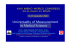### this product is designed for use in closed course racing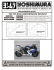### Intelligence in Living Cells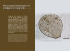### Troubleshooting Slow or No Treadle Movement### THIS PRODUCT IS DESIGNED FOR USE IN CLOSED### The 1960s: Pop, Op, and Minimalism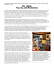### Acta Universitatis Lodziensis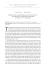### Cranial Nerves Atlas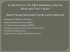### how to identify rhododendron and azalea problems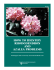### Lorenz system II - Harvard Mathematics Department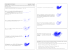### TreadClimber - Fitness 4 Home Superstore